Journal homepage ISSN: 1303 - 2968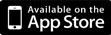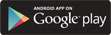©Journal of Sports Science and Medicine (2003) 02, 139 - 143 Research article A New Approach to Modeling Vertical Stiffness in Heel-Toe Distance Runners Iain Hunter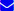Author Information Department of Physical Education, Brigham Young University, Provo, Utah, USA Iain Hunter✉ Brigham Young University, 120D Richards Building, Provo, UT 84602, USAEmail: iain_hunter@byu.edu Publish Date Received: 07-06-2003 Accepted: 10-10-2003 Published (online): 01-12-2003 × Iain Hunter. (2003) A New Approach to Modeling Vertical Stiffness in Heel-Toe Distance Runners. Journal of Sports Science and Medicine (02), 139 - 143.  Email link to this article × Iain Hunter. (2003) A New Approach to Modeling Vertical Stiffness in Heel-Toe Distance Runners. Journal of Sports Science and Medicine (02), 139 - 143. Your name: Your E-mail: Recipient’s E-mail:
 Abstract Introduction Methods Results Discussion Author biography References

ABSTRACT

Various models have been used to describe distance running technique. Among these, the mass-spring model is fairly simple to use and apply, but when employed as a model, does not predict vertical force accurately especially when a heel strike is exhibited. The purpose of this article is to demonstrate how the mass-spring model can be modified to provide a simple, yet accurate prediction of ground reaction forces in distance running. Sixteen subjects ran on a force instrumented treadmill. Vertical forces during running at a self-selected pace were collected at 500 Hz. Vertical stiffness was calculated using the conventional mass-spring model with a constant stiffness and then a high-low method where stiffness was varied from a high to low value during the heel strike. Fishers z-test was used to compare correlations between predicted and measured ground reaction forces for each method of calculating stiffness. The high-low method of calculating stiffness provided a better fit of predicted to measured ground reaction forces than the constant stiffness method (p < 0.01). The high-low method of calculating stiffness avoids the difficulties of applying multiple masses, springs, or dampers while simply, yet accurately matching predicted to measured ground reaction forces.

Key words: Leg stiffness, modeling, leg spring

Key Points

 INTRODUCTION

The mass-spring model provides much useful information when evaluating distance running technique. The model describes the body as a mass centered above a spring that is connected to the ground during the stance phase of running (Figure 1). It has been used to investigate energy cost of running, aerobic demand, speed of running, stride frequency, and how technique changes with various surfaces (McMahon and Cheng, 1990; Farley and Gonzalez, 1996; Heise and Martin, 1998; Dalleau et al., 1998; Arampatzis et al., 1999; Kerdok et al., 2002).

However, there are limitations dealing with the application of stiffness. When modeling a cycle of running with a mass-spring system, prediction of force versus time curves using a constant stiffness of the spring are clearly imperfect, especially when the runner exhibits a heel strike (Figure 2). The mass-spring model assumes a linear spring; however, in running the body does not act linearly. Prior to heel strike, runners’ bodies react more stiffly to the ground than after heel strike.

Adding to the difficulty of matching predicted versus measured ground reaction forces is the fact that much variation exists from person to person, with various speeds of running, stride rates, and running surfaces. Other models have attempted to more accurately reproduce the running motion (Nigg and Liu, 1999; Derrick et al., 2000). However, these models are quite complex requiring a number of variables to be defined and calculated. The large quantity of variables in these models makes statistical correlations with other aspects of running difficult to investigate.

A modification to the traditional mass-spring model was tested in this study to see whether it could better match predicted ground reaction forces to measured ground reaction forces. Since the body does not react as a linear spring during stance, application of a model with varying stiffness may be more appropriate. The initial impact during stance exhibits a relatively stiff reaction to the ground, followed by a more pliant reaction following heel strike. The model investigated in this study applies an initially high stiffness which drops to a lower stiffness, which it maintains following heel strike.

This article will show how applying a variable stiffness to the region around heel strike provides a much better fit of predicted to measured ground reaction forces. This will allow for a mass-spring model that will accurately model ground reaction forces using only two variables, high and low stiffnesses.

 METHODS

Subjects all signed an informed consent approved by the university’s internal review board. Nine men and seven women (age = 28 ± 6 yrs, height = 1.76 ± 0.10 m, mass = 70.1 ± 10.2 kg) ran at a self-selected pace on a force instrumented treadmill for 10 minutes allowing for sufficient warm-up as technique may change slightly during the first few minutes of running (Candau et al., 1998). During the ninth minute, vertical force data were collected at 500 Hz. These data were smoothed using a Butterworth filter set at 50 Hz. Average vertical stiffness was taken from ten consecutive right steps.

Constant stiffness

Vertical stiffness can be calculated by solving for k in the equation: F=ks.

Where F represents the ground reaction force after accounting for body weight, k represents the vertical stiffness, and s represents the vertical displacement of the center of mass. In this study, we divided vertical force by body weight to obtain center of mass accelerations. A double-integration of acceleration provided changes in center of mass position. Assuming the person lands at the same height for each step, the initial vertical velocity needed for the integration can be easily obtained when consecutive steps are recorded. The stiffness is then found by dividing peak vertical force by vertical displacement of the center of mass during stance (Farley and Gonzalez, 1996).

High-low stiffness

Vertical stiffness is typically measured using a constant k. However, this study applies a changing stiffness in order to more accurately model what occurs during stance. Initially, the body reacts relatively stiffly to the ground. Following heel strike, the body reacts more pliant. Four characteristics were used to calculate stiffness for the high-low method: initial or high stiffness, final or low stiffness, and two points in time representing a transition from the high to low stiffnesses. The smooth transition from high to low stiffness was created using half a cosine wave (Figure 3). High and low stiffnesses were determined by an optimal fit of measured to predicted force using the initial velocity and mass. The transition from high to low stiffness begins at the peak of heel strike and ends at the minimum of the valley immediately after heel strike (Figure 3). Outside of the transition, the modeled stiffness remains constant.

Vertical forces throughout stance can be predicted using the initial velocity, calculated stiffness, and body weight. Correlations were made between measured vertical force and predicted vertical force for the constant and high-low stiffness methods. Differences between correlations using the two methods were investigated using Fisher’s z-test for comparing correlations (alpha = 0.05).

 RESULTS

Fisher’s z-test for comparing correlations of measured to predicted vertical force showed a significant difference between the constant stiffness method and the high-low method (p < 0.01) (Table 1). Visually, the high-low method was able to closely match the heel strike, which the constant stiffness method was unable to do at all (Figure 3). The high-low method also visually matched the difference in slopes of force versus time up to heel strike and following the active peak much better than the constant stiffness method.

 DISCUSSION

For the mass-spring model to be useful in describing running technique, the predicted force based upon the model should match the measured force as closely as possible. The heel strike and hysteresis of a force versus center of mass displacement curve shown in figure 4 are a major part of the problem with the traditional mass-spring model (Dutto and Smith, 2002). The high-low stiffness mass-spring model provides more accurate predictions of vertical ground reaction forces than a constant stiffness model. The varying stiffness even closely matches the heel strike. This model provides a simple, but relatively accurate representation of the body during distance running.

The high-low stiffness model requires two variables, creating a difficulty in making correlations between vertical stiffness and other characteristics of running such as stride length and rate, running surface, footwear, and anthropometric variables. However, other alternatives to the complete high-low stiffness model are possible. The initial stiffness in this model represents the high value of stiffness immediately after ground contact. The final stiffness represents the stiffness following heel strike. Using these two characteristics individually may be useful in determining correlations with other running characteristics without using the entire model.

Vertical stiffness varies with running surface (Ferris and Farley, 1997; Ferris et al., 1998; Farley et al., 1998; Ferris et al., 1999). The variability added by surface will likely add to inaccuracies of the traditional mass-spring model. Vertical stiffness also changes with fatigue, speed, and stride frequency (McMahon and Cheng, 1990; Farley et al., 1991; Farley and Gonzalez, 1996; Dutto and Smith, 2002). Since the high-low model adjusts for variations in heel-strike and the hysteresis of the force versus displacement curve, it should provide a better fit of predicted versus measured ground reaction forces for a variety of situations.

 CONCLUSION

The high-low stiffness method of modeling the body in distance running matches predicted ground reaction forces to measured ground reaction forces much better than the constant stiffness method. Since the high-low method produces a better fit, it may be more useful in correlating characteristics of running with vertical stiffness. One disadvantage to implementing the high-low method is that two variables are required to describe stiffness during the stance phase of running. However, there may be useful application to using just one of these variables for certain investigations.

AUTHOR BIOGRAPHYIain Hunter Employment: Ass. Prof. at Brigham Young University, USA Degree: PhD Research interests: Distance running economy and technique of track and field events. E-mail: iain_hunter@byu.edu

REFERENCES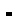Arampatzis A., Bruggemann G.P., Metzler V. (1999) The effect of speed on leg stiffness and joint kinetics in human running. Journal of Biomechanics 32, 1349-1353.Candau R., Belli A., Millet G. Y., Georges D., Barbier B., Rouillon J. D. (1998) Energy cost and running mechanics during a treadmill run to voluntary exhaustion in humans. European Journal of Applied Physiology and Occupational Physiology 77, 479-485.Dalleau G., Belli A., Bourdin M., Lacour J. R. (1998) The spring-mass model and the energy cost of treadmill running. European Journal of Applied Physiology and Occupational Physiology 77, 257-263.Derrick T. R., Caldwell G. E., Hamill J. (2000) Modelling the stiffness characteristics of the human body while running with various stride lengths. Journal of Applied Biomechanics 16, 36-51.Dutto D. J., Smith G. A. (2002) Changes in spring-mass characteristics during treadmill running to exhaustion. Medicine and Science in Sports and Exercise 34, 1324-1331.Farley C. T., Blickhan R., Saito J., Taylor C. R. (1991) Hopping frequency in humans: a test of how springs set stride frequency in bouncing gaits. Journal of Applied Physiology 71, 2127-2132.Farley C. T., Gonzalez O. (1996) Leg stiffness and stride frequency in human running. Journal of Biomechanics 29, 181-186.Farley C. T., Houdijk H. H., Van Strien C., Louie M. (1998) Mechanism of leg stiffness adjustment for hopping on surfaces of different stiffnesses. Journal of Applied Physiology 85, 1044-1055.Ferris D. P., Farley C. T. (1997) Interaction of leg stiffness and surface stiffness during human hopping. Journal of Applied Physiology 82, 15-22.Ferris D. P., Liang K., Farley C. T. (1999) Runners adjust leg stiffness for their first step on a new running surface. Journal of Biomechanics 32, 787-794.Ferris D. P., Louie M., Farley C. T. (1998) Running in the real world: adjusting leg stiffness for different surfaces. Proceedings of the Royal Society of London: Biological Sciences 265, 989-994.Heise G. D., Martin P. E. (1998) "Leg spring ”characteristics and the aerobic demand of running. Medicine and Science in Sports and Exercise 30, 750-754.Kerdok A. E., Biewener A. A., McMahon T. A., Weyand P. G., Herr H. M. (2002) Energetics and mechanics of human running on surfaces of different stiffnesses. Journal of Applied Physiology 92, 469-478.McMahon T. A., Cheng G. C. (1990) The mechanics of running: How does stiffness couple with speed. Journal of Biomechanics 23, 65-78.Nigg B. M., Liu W. (1999) The effect of muscle stiffness and damping on simulated impact force peaks during running. Journal of Biomechanics 32, 849-856.

 Home Issues About Authors Contact Current Editorial board Authors instructions Email alerts In Press Mission For Reviewers Archive Scope Supplements Statistics Most Read Articles Most Cited Articles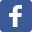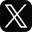This work is licensed under a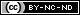Creative Commons Attribution-NonCommercial-NoDerivatives 4.0 International License.